# Velocity control exampleusing Drotek’s L6234 driver

Drotek’s L6234 breakout board is a very minimalistic 3-phase BLDC motor driver and is very suitable for jump-starting your FOC experience. Here we the velocity control example project using the SimpleFOClibrary and this hardware:

Arduino UNODrotek L6234 breakout boardAMT 103 encoderIPower GBM8017-120T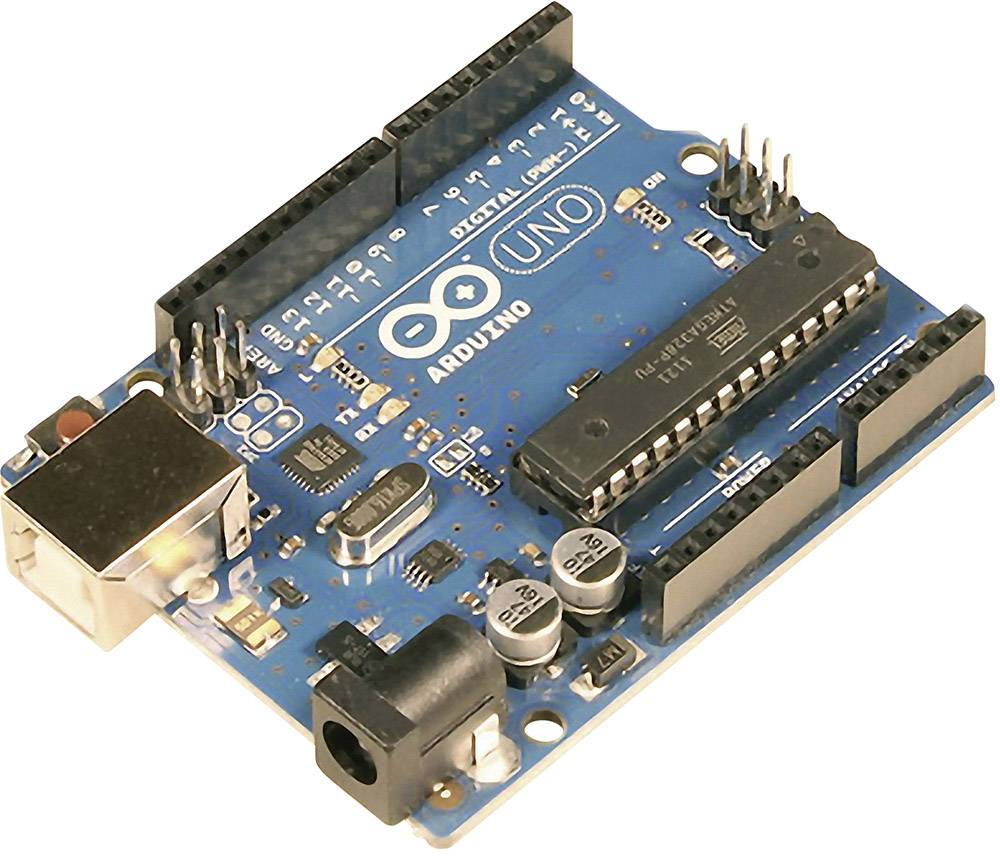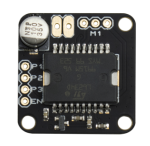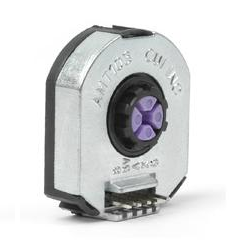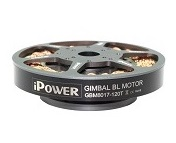# Connecting everything together

For a bit more in depth explanation of Arduino UNO and L6234 connection please check the connection examples.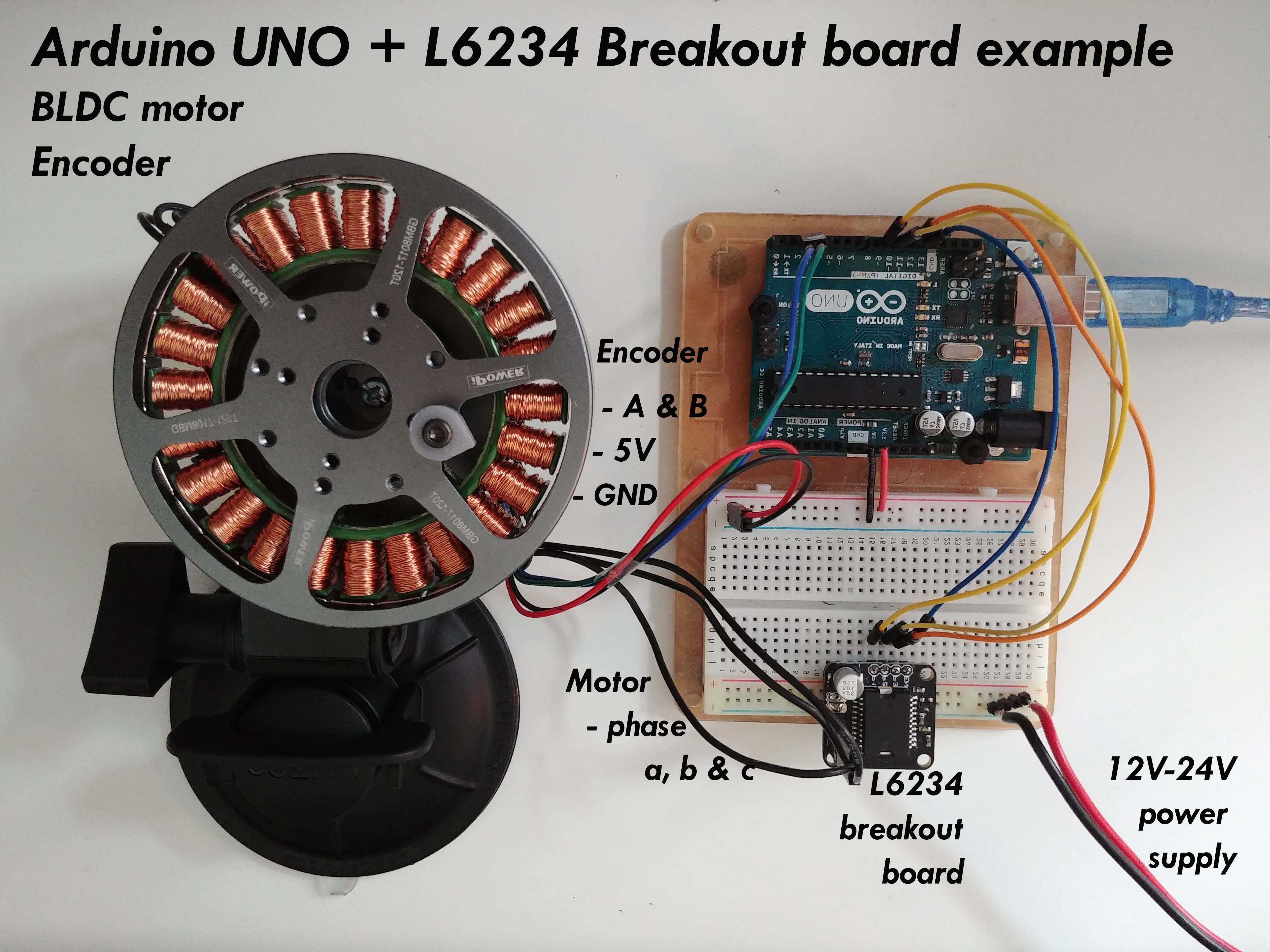### Encoder

• Encoder channels `A` and `B` are connected to the Arduino’s external interrupt pins `2` and `3`.

### L6234 breakout board

• Connected to the arduino pins `9`,`10` and `11` (you can use also pins `5` and `6`).
• Additionally you can connect the `enable` pin to the any digital pin of the arduino the picture shows pin `8` but this is optional. You can connect the driver enable directly to 5v.
• Make sure you connect the common ground of the power supply and your Arduino

### Motor

• Motor phases `a`, `b` and `c` are connected directly to the driver outputs

# Arduino code

Let’s go through the full code for this example and write it together. First thing you need to do is include the `SimpleFOC` library:

``````#include <SimpleFOC.h>
``````

Make sure you have the library installed. If you still don’t have it please check the get started page

## Encoder code

First we define the `Encoder` class with the A and B channel pins and number of impulses per revolution.

``````// define Encoder
Encoder encoder = Encoder(2, 3, 2048);
``````

Then we define the buffering callback functions.

``````// channel A and B callbacks
void doA(){encoder.handleA();}
void doB(){encoder.handleB();}
``````

In the `setup()` function we initialize the encoder and enable interrupts:

``````// initialize encoder hardware
encoder.init();
// hardware interrupt enable
encoder.enableInterrupts(doA, doB);
``````

And that is it, let’s setup the motor.

For more configuration parameters of the encoders please check the `Encoder` class docs.

## Motor code

First we need to define the `BLDCMotor` class with the number od pole pairs (`14`)

``````// define BLDC motor
BLDCMotor motor = BLDCMotor(14);
``````
If you are not sure what your pole pairs number is please check the `find_pole_pairs.ino` example.

Next we need to define the `BLDCDriver3PWM` class with the PWM pin numbers of the motor and the driver enable pin

``````// define BLDC driver
BLDCDriver3PWM driver = BLDCDriver3PWM(9, 10, 11, 8);
``````

Then in the `setup()` we configure first the voltage of the power supply if it is not `12` Volts and init the driver.

``````// power supply voltage
// default 12V
driver.voltage_power_supply = 12;
driver.init();
``````

Then we tell the motor which control loop to run by specifying the `motor.controller` variable.

``````// set control loop type to be used
// MotionControlType::torque
// MotionControlType::velocity
// MotionControlType::angle
motor.controller = MotionControlType::velocity;
``````

Now we configure the PI controller parameters

``````// velocity PI controller parameters
// default P=0.5 I = 10
motor.PID_velocity.P = 0.2;
motor.PID_velocity.I = 20;
//default voltage_power_supply
motor.voltage_limit = 6;
``````

Additionally we can configure the Low pass filter time constant `Tf`

``````// velocity low pass filtering
// default 5ms - try different values to see what is the best.
// the lower the less filtered
motor.LPF_velocity.Tf = 0.01;
``````

And finally we connect the encoder and the driver to the motor, do the hardware init and init of the Field Oriented Control.

``````// link the motor to the sensor

// initialize motor
motor.init();
// align encoder and start FOC
motor.initFOC();
``````

The last peace of code important for the motor is of course the FOC routine in the `loop` function.

``````void loop() {
// iterative FOC function
motor.loopFOC();

// iterative function setting and calculating the velocity loop
// this function can be run at much lower frequency than loopFOC function
motor.move(target_velocity);
}
``````

That is it, let’s see the full code now!

For more configuration parameters and control loops please check the `BLDCMotor` class doc.

## Full Arduino code

To the full code I have added a small serial commander interface, to be able to change velocity target value in real time.

``````#include <SimpleFOC.h>

// define BLDC motor
BLDCMotor motor = BLDCMotor( 14 );
BLDCDriver3PWM driver = BLDCDriver3PWM(9, 10, 11, 8);

// define Encoder
Encoder encoder = Encoder(2, 3, 2048);
// channel A and B callbacks
void doA(){encoder.handleA();}
void doB(){encoder.handleB();}

// velocity set point variable
float target_velocity = 0;
// commander interface
Commander command = Commander(Serial);
void onTarget(char* cmd){ command.scalar(&target_velocity, cmd); }

void setup() {

// initialize encoder hardware
encoder.init();
// hardware interrupt enable
encoder.enableInterrupts(doA, doB);

// power supply voltage
// default 12V
driver.voltage_power_supply = 12;
driver.init();
// link the motor to the driver

// set control loop type to be used
motor.controller = MotionControlType::velocity;

// velocity PI controller parameters
// default P=0.5 I = 10
motor.PID_velocity.P = 0.2;
motor.PID_velocity.I = 20;
//default voltage_power_supply
motor.voltage_limit = 6;

// velocity low pass filtering
// default 5ms - try different values to see what is the best.
// the lower the less filtered
motor.LPF_velocity.Tf = 0.01;

// link the motor to the sensor

// initialize motor
motor.init();
// align encoder and start FOC
motor.initFOC();

// monitoring port
Serial.begin(115200);
Serial.println("Set the target velocity using serial terminal:");
_delay(1000);
}

void loop() {
// iterative foc function
motor.loopFOC();

// iterative function setting and calculating the velocity loop
// this function can be run at much lower frequency than loopFOC function
motor.move(target_velocity);

// user communication
command.run();
}
``````